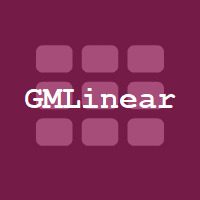# GMLinear

#### FrostyCat

You must be logged in to obtain assets

### Description

Overview

GMLinear is an implementation of matrix and vector operations in pure GML. You can use it to simplify many common calculations in 2D and 3D geometry and implement algorithms/formulas involving linear algebra. Hard-coded optimized functions are available for 2D, 3D and 4D vectors and 2x2, 3x3 and 4x4 matrices.

Documentation

Available on GitHub in PDF form: v2.0.0 Function Listings

Examples

``````vec1_3d = [3, 4, 5];
vec2_3d = [1, 2, 3];
mat_3x3 = r33(
1, 0, 0,
0, 0, 1,
0, 1, 0
);
vec_sum = r3_add(vec1_3d, vec2_3d); //[4, 6, 8]
dot_prod = r3_dot(vec1_3d, vec2_3d); //26
cross_prod = r3_cross(vec1_3d, vec2_3d); //[2, -4, 2]
vec_mult = r33_transform(mat_3x3, vec1_3d); //[3, 5, 4]
``````

GMLinear is 100% open source!

Bug reports and contributions welcome on the official GitHub repository.